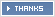# Waec 2018 Physics Practical Answers – May/June Expo(1a)
TABULATE:
S/N: 1, 2 , 3, 4, 5
L(cm): 90.00, 80.00, 70.00, 60.00, 50.00
T(s): 38.00, 36.00, 34.00, 31.00, 28.00
T = t/20(sqt): 1.90, 1.80, 1.70, 1.55, 1.40
√L(cm^1/2): 9.49, 8.94, 8.37, 7.73, 7.07

(1aix)
Slope(s) = Δvertical/Δhorizontal
=1.90 – 1.55/9.49 – 7.75
= 0.35/1.74
S = 0.201

(1ax)
g = 4π²/S²
= 4 ×(3.142)²/(0.201)²
= 977.4cm/s²

(1axi)
(i) I ensured the angle of oscillation is relatively small.
(ii) I ensured that no external force is added to the system of oscillation.
(iii) I ensured the oscillation is perfect

(2ai)
fo=15cm
2av)
a=60.00cm
b=20.00cm
Hence L=a/b=60.00/20.00
L=3

(2avi)
TABULATE
S/N:1,2,3,4,5
b(cm):20.00,25.00,35.00,40.00
a(cm):60.00,37.50,30.00,26.25,24.00
L=a/b:3.00,1.50,1.00,0.75,0.60
2avii)
Slope=Change in L/Change in a
=(3-0.25)/(60-18.6)
=2.75/41.4
=0.006642
2aviii)
S^-1=1/S
=(1/0.0066425cm)
S=15.05
S=15cm

(2aix)
PRECAUTIONS
-I ensured that all apparatus are in straight line
-I avoided error due to parallax when reading the metre rule
-I avoided zero error on the metre rule

(2bi)
u=10cm
f=15cm
Using 1/v+1/u=1/f
1/f-1/u=1/v
1/v=1/15-1/10
1/v=(2-3)/30
1/v=-1/30
v=-30cm
The characteristics of image formed are:
-It is virtual
-It is enlarged and magnified ie twice or two times as big as the object m=2

(2bii)
The concave mirror mounted in its holder is moved to and fro in front of the screen until a sharp image of the cross wire of the ray box is formed on the screen adjacent of the object.The distance between the mirror and the screen was measured as 30cm since the radius of curvature r=2fo then half is distance

(2bi)
The characteristics of imaged formed are :
i)It is virtual
ii) It is enlarged or magnified i.e. twice or two times bigger as the object(m=2)

(3a)
TABULATE:
x(cm):10,20,30,40,50,60
V(v):0.65,0.75,1.00,1.20,1.45,1.55
I(A):0.20,0.30,0.35,0.40,0.45,0.55
LogV(v):-0.187,-0.125,0.000,0.079,0.161,0.190
LogI(ohm):-0.699,-0.523,-0.456,-0.396,-0.391,-0.360
SLOPE(s):=( LogI2-LOgI1)/(LogV2-LogV1)
=-0.2-(-0.7)/0.3-(-0.2)
=0.5/0.5
=1AV^-1
(3axi)
(i) I ensured neat and tight terminals
(ii) I opened the key when reading was taken

(3bi)
(i)The brightness of the bulb increases
(ii) The voltage and current through the bulb increases

(3bii)
(i) diode
(ii) transistor

NOTE!!!
Always subscribe early!!!:-1. Plz I Need Physic ans

2. Pls i need waec geography essay and objective, questions and answers before the exam commence

3. April 4, 2018 at 8:14 am

Physics practical

4. Bhh

5. Pls Send Me Physics Pratical Answer B4 D Commencement Of Exam

• Okay